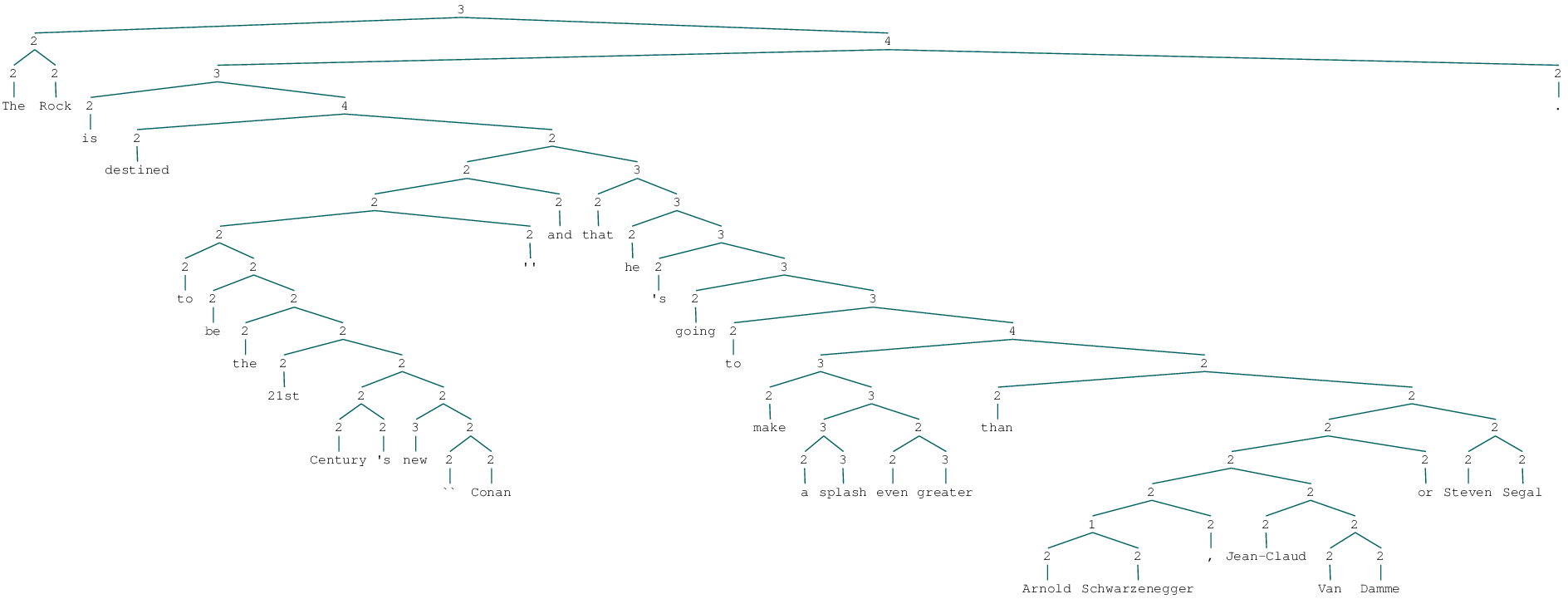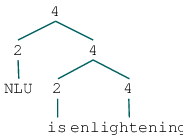Supervised sentiment: overview of the Stanford Sentiment Treebank¶

In :
__author__ = "Christopher Potts"
__version__ = "CS224u, Stanford, Spring 2020"

Overview of this unit¶

We have a few inter-related goals for this unit:

• Provide a basic introduction to supervised learning in the context of a problem that has long been central to academic research and industry applications: sentiment analysis.

• Explore and evaluate a diverse array of methods for modeling sentiment:

• Hand-built feature functions with (mostly linear) classifiers
• Dense feature representations derived from VSMs as we built them in the previous unit
• Recurrent neural networks (RNNs)
• Tree-structured neural networks
• Begin discussing and implementing responsible methods for hyperparameter optimization and classifier assessment and comparison.

The unit is built around the Stanford Sentiment Treebank (SST), a widely-used resource for evaluating supervised NLU models, and one that provides rich linguistic representations.

Paths through the material¶

• If you're relatively new to supervised learning, we suggest studying the details of this notebook closely and following the links to additional resources.

• If you're familiar with supervised learning, then you can focus right away on innovative feature representations and modeling.

• As of this writing, the state-of-the-art for the SST seems to be around 88% accuracy for the binary problem and 48% accuracy for the five-class problem. Perhaps you can best these numbers!

Overview of this notebook¶

This is the first notebook in this unit. It does two things:

• Introduces sentiment analysis as a task.
• Introduces the SST and our tools for reading that corpus.

The complexity of sentiment analysis¶

Sentiment analysis seems simple at first but turns out to exhibit all of the complexity of full natural language understanding. To see this, consider how your intuitions about the sentiment of the following sentences can change depending on perspective, social relationships, tone of voice, and other aspects of the context of utterance:

1. There was an earthquake in LA.
2. The team failed the physical challenge. (We win/lose!)
3. They said it would be great. They were right/wrong.
4. Many consider the masterpiece bewildering, boring, slow-moving or annoying.
5. The party fat-cats are sipping their expensive, imported wines.
6. Oh, you're terrible!

SST mostly steers around these challenges by including only focused, evaluative texts (sentences from movie reviews), but you should have them in mind if you consider new domains and applications for the ideas.

Set-up¶

• Make sure your environment includes all the requirements for the cs224u repository.

• If you haven't already, download the course data, unpack it, and place it in the directory containing the course repository – the same directory as this notebook. (If you want to put it somewhere else, change SST_HOME below.)

In :
from nltk.tree import Tree
import os
import pandas as pd
import sst
In :
SST_HOME = os.path.join('data', 'trees')

• The train/dev/test SST distribution contains files that are lists of trees where the part-of-speech tags have been replaced with sentiment scores 0...4:

• 0 and 1 are negative labels.
• 2 is a neutral label.
• 3 and 4 are positive labels.
• Our readers are iterators that yield (tree, label) pairs, where tree is an NLTK Tree instance and score is a string.

We'll mainly work with sst.train_reader and sst.dev_reader.

In :
tree, score = next(sst.train_reader(SST_HOME))

Here, score is one of the labels. tree is an NLTK Tree instance. It should render pretty legibly in your browser:

In :
tree
Out:This is what it actually looks like, of course:

In :
(tree,)
Out:
(Tree('3', [Tree('2', [Tree('2', ['The']), Tree('2', ['Rock'])]), Tree('4', [Tree('3', [Tree('2', ['is']), Tree('4', [Tree('2', ['destined']), Tree('2', [Tree('2', [Tree('2', [Tree('2', [Tree('2', ['to']), Tree('2', [Tree('2', ['be']), Tree('2', [Tree('2', ['the']), Tree('2', [Tree('2', ['21st']), Tree('2', [Tree('2', [Tree('2', ['Century']), Tree('2', ["'s"])]), Tree('2', [Tree('3', ['new']), Tree('2', [Tree('2', ['']), Tree('2', ['Conan'])])])])])])])]), Tree('2', ["''"])]), Tree('2', ['and'])]), Tree('3', [Tree('2', ['that']), Tree('3', [Tree('2', ['he']), Tree('3', [Tree('2', ["'s"]), Tree('3', [Tree('2', ['going']), Tree('3', [Tree('2', ['to']), Tree('4', [Tree('3', [Tree('2', ['make']), Tree('3', [Tree('3', [Tree('2', ['a']), Tree('3', ['splash'])]), Tree('2', [Tree('2', ['even']), Tree('3', ['greater'])])])]), Tree('2', [Tree('2', ['than']), Tree('2', [Tree('2', [Tree('2', [Tree('2', [Tree('1', [Tree('2', ['Arnold']), Tree('2', ['Schwarzenegger'])]), Tree('2', [','])]), Tree('2', [Tree('2', ['Jean-Claud']), Tree('2', [Tree('2', ['Van']), Tree('2', ['Damme'])])])]), Tree('2', ['or'])]), Tree('2', [Tree('2', ['Steven']), Tree('2', ['Segal'])])])])])])])])])])])])]), Tree('2', ['.'])])]),)

Here's a smaller example:

In :
Tree.fromstring("""(4 (2 NLU) (4 (2 is) (4 enlightening)))""")
Out:Methodological notes¶

• We've deliberately ignored test readers. We urge you not to use the test set until and unless you are running experiments for a final project or similar. Overuse of test-sets corrupts them, since even subtle lessons learned from those runs can be incorporated back into model-building efforts.

• We actually have mixed feelings about the overuse of dev that might result from working with these notebooks! We've tried to encourage using just splits of the training data for assessment most of the time, with only occasionally use of dev. This will give you a clearer picture of how you will ultimately do on test; over-use of dev can lead to over-fitting on that particular dataset with a resulting loss of performance of test.

Modeling the SST labels¶

Working with the SST involves making decisions about how to handle the raw SST labels. The interpretation of these labels is as follows (Socher et al., sec. 3):

• '0': very negative
• '1': negative
• '2': neutral
• '3': positive
• '4': very positive

The labels look like they could be treated as totally ordered, even continuous. However, conceptually, they do not form such an order. Rather, they consist of three separate classes, with the negative and positive classes being totally ordered in opposite directions:

• '0' > '1': negative
• '2': neutral
• '4' > '3': positive

Thus, in this notebook, we'll look mainly at binary (positive/negative) and ternary tasks.

A related note: the above shows that the fine-grained sentiment task for the SST is particularly punishing as usually formulated, since it ignores the partial-order structure in the categories completely. As a result, mistaking '0' for '1' is as bad as mistaking '0' for '4', though the first error is clearly less severe than the second.

The functions sst.binary_class_func and sst.ternary_class_func will convert the labels for you, and recommended usage is to use them as the class_func keyword argument to train_reader and dev_reader; examples below.

Train label distributions¶

Check that these numbers all match those reported in Socher et al. 2013, sec 5.1.

In :
train_labels = [y for tree, y in sst.train_reader(SST_HOME)]
In :
print("Total train examples: {:,}".format(len(train_labels)))
Total train examples: 8,544

Distribution over the full label set:

In :
pd.Series(train_labels).value_counts()
Out:
3    2322
1    2218
2    1624
4    1288
0    1092
dtype: int64

Binary label conversion:

In :
binary_train_labels = [
y for tree, y in sst.train_reader(SST_HOME, class_func=sst.binary_class_func)]
In :
print("Total binary train examples: {:,}".format(len(binary_train_labels)))
Total binary train examples: 6,920
In :
pd.Series(binary_train_labels).value_counts()
Out:
positive    3610
negative    3310
dtype: int64

Ternary label conversion:

In :
ternary_train_labels = [
y for tree, y in sst.train_reader(SST_HOME, class_func=sst.ternary_class_func)]

pd.Series(ternary_train_labels).value_counts()
Out:
positive    3610
negative    3310
neutral     1624
dtype: int64

Dev label distributions¶

Check that these numbers all match those reported in Socher et al. 2013, sec 5.1.

In :
dev_labels = [y for tree, y in sst.dev_reader(SST_HOME)]
In :
print("Total dev examples: {:,}".format(len(dev_labels)))
Total dev examples: 1,101
In :
pd.Series(dev_labels).value_counts()
Out:
1    289
3    279
2    229
4    165
0    139
dtype: int64

Binary label conversion:

In :
binary_dev_labels = [
y for tree, y in sst.dev_reader(SST_HOME, class_func=sst.binary_class_func)]
In :
print("Total binary dev examples: {:,}".format(len(binary_dev_labels)))
Total binary dev examples: 872
In :
pd.Series(binary_dev_labels).value_counts()
Out:
positive    444
negative    428
dtype: int64

Ternary label conversion:

In :
ternary_dev_labels = [
y for tree, y in sst.dev_reader(SST_HOME, class_func=sst.ternary_class_func)]

pd.Series(ternary_dev_labels).value_counts()
Out:
positive    444
negative    428
neutral     229
dtype: int64